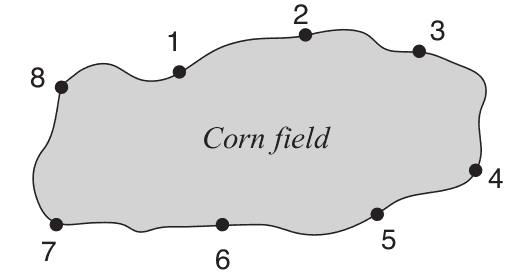### F - Farm robot

##### Languages: C, C++, Java, JavaScript, ... (details)

To discourage birds such as crows and sparrows from feeding on his crops a farmer needed to put some scarecrows in his corn field. His nephew really likes robots, and suggested that he should use a robot scarecrow instead: "A single robot scarecrow can better protect the whole corn field and will last way more than ten traditional ones!", he said.

Since the farmer thinks his nephew is a smart boy, he took his advice and bought a robot scarecrow. The robot moves along a pathway that surrounds the corn field. In the pathway there are $N$ unmanned charging stations, numbered sequentially in clockwise order starting from $1$. The figure below shows an example with eight charging stations.The robot begins every day at station number $1$, and is issued a sequence of commands that are to be performed in order during the day. These commands are generated based on advanced machine learning algorithms that work on data collected by sensors spread through the corn field, ensuring an optimal coverage of the crop. Each command results in the robot moving to another charging station next to the one it is currently at, either in clockwise or counter-clockwise direction.

Despite the promises of optimal coverage by the robot, at the end of a certain day the farmer found part of his crop devastated. To figure out what might have happened the farmer wants to know how many times the robot was at the charging station closest to the devastated area. Given the number of the station closest to the devastated area and the sequence of commands for a single day, can you help the farmer find this number?

#### Input

The first line contains three integers $N$, $C$ and $S$ representing respectively the number of posts ($2 \le N \le 100$), the number of commands ($1 \le C \le 1000$) and the charging station closest to the devastated area ($1 \le S \le N$). The second line contains $C$ integers $X_1, X_2, \dots, X_C$, representing the sequence of commands received by the robot scarecrow. For $i = 1, 2, \dots, C$, if $X_i$ is $1$ then the $i$-th command means "move to the next charging station in clockwise order", whereas if $X_i$ is $-1$ then the $i$-th command means "move to the next charging station in counter-clockwise order". The robot always starts at station
number $1$.

#### Output

Output a line with an integer representing the number of times the robot was at station number $S$ during the day.

#### Sample test(s)

Input
8 8 3 1 -1 1 1 1 -1 1 1
Output
2
Input
5 4 1 1 1 1 1
Output
1
Input
2 1 1 1
Output
1
Input
2 1 2 1
Output
1
Input
2 2 1 -1 1
Output
2
Input
2 2 1 -1 -1
Output
2